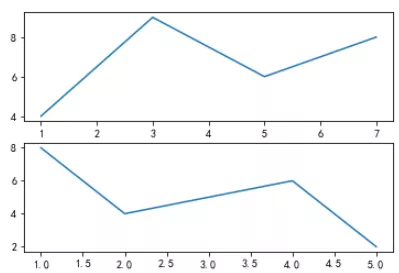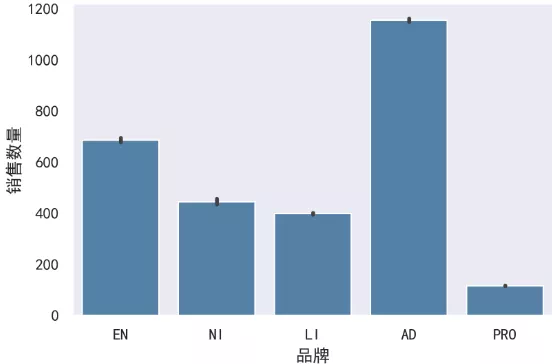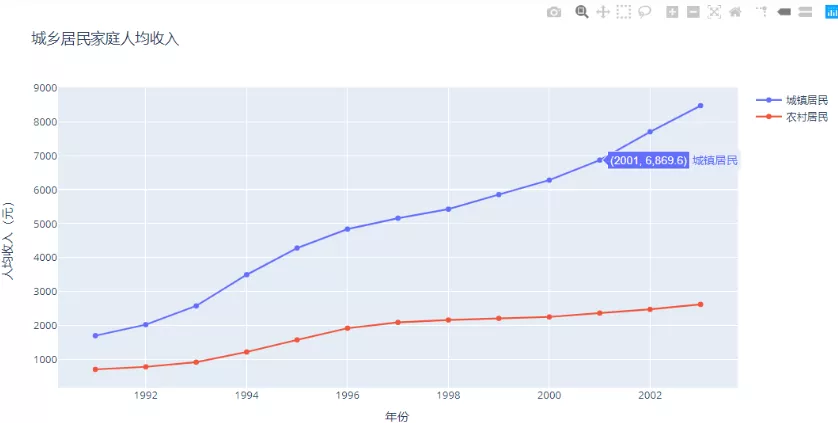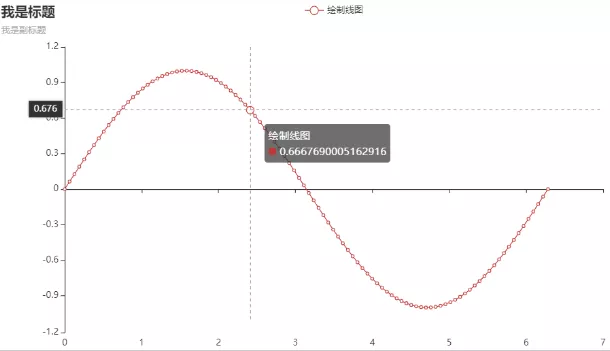# Python 常用绘图库原理及示例

Python绘图库太多不知道学哪一个？

## 1 matplotlib绘图原理

matplotlib绘图原理：http://suo.im/678FCo

1）绘图原理说明

① 导库;
② 创建figure画布对象;
③ 获取对应位置的axes坐标系对象;
④ 调用axes对象，进行对应位置的图形绘制;
⑤ 显示图形;

2）案例说明

``````# 1.导入相关库
import matplotlib as mpl
import matplotlib.pyplot as plt
# 2.创建figure画布对象
figure = plt.figure()
# 3.获取对应位置的axes坐标系对象
# 4.调用axes对象，进行对应位置的图形绘制
axes1.plot([1,3,5,7],[4,9,6,8])
axes2.plot([1,2,4,5],[8,4,6,2])
# 5.显示图形
figure.show()``````## 2 seaborn绘图原理

seaborn是matplotlib的更高级的封装。因此学习seaborn之前，首先要知道matplotlib的绘图原理。由于seaborn是matplotlib的更高级的封装，对于matplotlib的那些调优参数设置，也都可以在使用seaborn绘制图形之后使用。

seaborn绘图原理：http://suo.im/5D3VPX

1）案例说明

``````# 1.导入相关库
import seaborn as sns
import matplotlib.pyplot as plt

sns.set_style("dark")
plt.rcParams["font.sans-serif"] = ["SimHei"]
plt.rcParams["axes.unicode_minus"] = False
# 注意：estimator表示对分组后的销售数量求和。默认是求均值。
sns.barplot(x="品牌",y="销售数量",data=df,color="steelblue",orient="v",estimator=sum)
plt.show()``````## 3. plotly绘图原理

plotly是一个基于javascript的绘图库，plotly绘图种类丰富，效果美观；

ploty默认的绘图结果，是一个HTML网页文件，通过浏览器可以直接查看；

plotly绘图原理：http://suo.im/5vxNTu

1）绘图原理说明

① 绘制图形轨迹，在ployly里面叫做trace，每一个轨迹是一个trace。

② 将轨迹包裹成一个列表，形成一个“轨迹列表”。一个轨迹放在一个列表中，多个轨迹也是放在一个列表中。

③ 创建画布的同时，并将上述的轨迹列表，传入到Figure()中。

④ 使用Layout()添加其他的绘图参数，完善图形。

⑤ 展示图形。

2）案例说明

``````import numpy as np
import pandas as pd
import plotly as py
import plotly.graph_objs as go
import plotly.expression as px
from plotly import tools

# 1.绘制图形轨迹，在ployly里面叫做`trace`，每一个轨迹是一个trace。
trace0 = go.Scatter(x=df["年份"],y=df["城镇居民"],name="城镇居民")
trace1 = go.Scatter(x=df["年份"],y=df["农村居民"],name="农村居民")
# 2.将轨迹包裹成一个列表，形成一个“轨迹列表”。一个轨迹放在一个列表中，多个轨迹也是放在一个列表中。
data = [trace0,trace1]
# 3.创建画布的同时，并将上述的`轨迹列表`，传入到`Figure()`中。
fig = go.Figure(data)
# 4.使用`Layout()`添加其他的绘图参数，完善图形。
fig.update_layout(
title="城乡居民家庭人均收入",
xaxis_title="年份",
yaxis_title="人均收入（元）"
)
# 5.展示图形。
fig.show()``````## 4 pyecharts绘图原理

Echarts是一个由百度开源的数据可视化工具，凭借着良好的交互性，精巧的图表设计，得到了众多开发者的认可。而python是一门富有表达力的语言，很适合用于数据处理。当数据分析遇上了数据可视化时，pyecharts诞生了。

pyecharts分为v0.5和v1两个大版本，v0.5和v1两个版本不兼容，v1是一个全新的版本，因此我们的学习尽量都是基于v1版本进行操作。

pyecharts的绘图原理：http://suo.im/5S1PF1

1）绘图原理说明

① 选择图表类型；

② 声明图形类并添加数据；

③ 选择全局变量；

④ 显示及保存图表；

2）案例说明

``````# 1.选择图表类型：我们使用的是线图，就直接从charts模块中导入Line这个模块；
from pyecharts.charts import Line
import pyecharts.options as opts
import numpy as np

x = np.linspace(0,2 * np.pi,100)
y = np.sin(x)

(
# 2.我们绘制的是Line线图，就需要实例化这个图形类，直接Line()即可；
Line()
# 3.添加数据，分别给x,y轴添加数据；
tooltip_opts=opts.TooltipOpts())
).render_notebook() # 4.render_notebook()用于显示及保存图表；``````